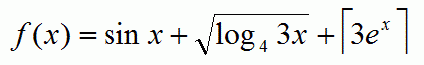Competitions

# Function

Let function f(x) is defined asCalculate the value of f(x) for a given x.

#### Input

Each line contains one double value x (x1).

#### Output

For each value x print on a separate line the value f(x) with 6 digits after the decimal point.

Time limit 1 second
Memory limit 128 MiB
Input example #1
1
2.3
2.56
7.123456

Output example #1
10.731685
31.926086
40.762019
3725.231017